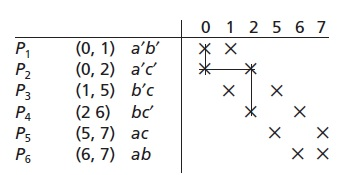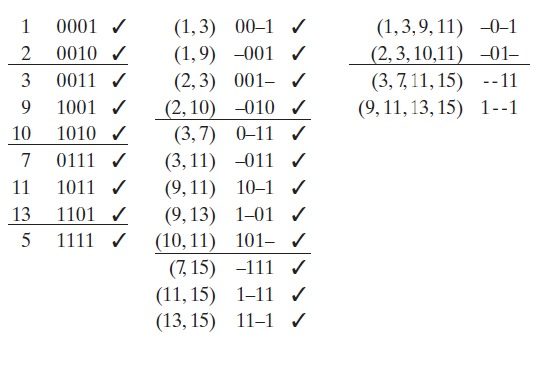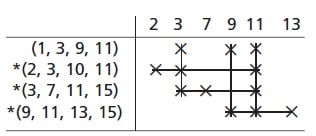Technical Article

# Prime Implicant Simplification Using Petrick’s Method

February 17, 2016 by Donald Krambeck

## This article follows the Quine McCluskey method article. We will now finding essential prime implicants using Petrick's method, simplifying incompletely specified functions, and using map-entered variables.

What is Petrick's Method?

Petrick's Method is used for determining minimum sum-of-product (SOP) solutions in a given prime implicants chart. Petrick's Method is another method used in Boolean Algebra, a simplification method used for complex circuits. Illustrated in Table 1.1 is an example that has two minimum solutions. If the number of variables increase in a given function, the number of prime implicants and complexity of the prime chart can increase drastically. In these types of cases, trial and error might be the best bet to find all of the minimum solution(s). The method mentioned in the previous article was a good way to find all the minimum solutions from a chart, but Petrick's method has a much better systematic approach. Before attempting to execute Petrick's method, all of the essential prime implicants; as well as the minterms they are covering need to be taken out of the prime implicants chart.Table 1.1

To grasp this concept fully, Table 1.1 will be used to illustrate Petrick's method. To start, each row on the table (P1P2P3) will need to be labeled. A logic function, P, will be formed, which holds true when all minterms have been covered up. This allows P1 to be a logic variable, which is true if the prime implicants in the P1 row are included in the solution. P2 should also be a logic variable, which is true when the prime implicants in the P2 row are included in the solution, etc. Looking at column 0, it appears that this column has X's in rows Pand P2; so rows P1 and Pmust be chosen to cover the minterm 0. Now, in order to cover the next minterm, minterm 1, rows Por P1 must be chosen; therefore, (PP3) must hold to be true. Likewise, in order to cover minterm 2, (P2 and P4) must also hold true. To easily cover the minterms 5, 6, and 7, expression (P3 + P5), (P4 + P6), and (P5 + P6) must hold to be true. Since all of the minterms need to be covered in order apply Petrick's method, the function below must hold true:

P = (P1 + P2)(P1 + P3)(P2 + P4)(P3 + P5 )(P4 + P6)(P5 + P6) = 1, What this means is that rows Por Pneed to be chosen, and row P1 or P3and row P2 or P4, et al.

Next, P needs to be completely reduced to a minimum SOP. This is relatively easy due to the fact that there are no compliments of the expression. After multiplying out, using the Boolean algebra rule (Y)(X + Z) = YZ, as well as the distributive law, the function below is as follows:

P = (P1 + P2P3)(P4 + P2P6)(P5 + P3P6)

= (P1P4 + P1P2P6 + P2P3PP2P3P6)(P5 + P3P6)

P1P4PP1P2P5PP2P3P4P5 + P2P3P5P6 + P1P3P4P6 + P1P2P3P6 + P2P3P4P6 + P2P3P6

After this, using XY to remove redundant terms from the logic function P, the following function is produced:

P = P1P4P5 + P1P2P5P6 + P2P3P4P5 + P1P3P4P6 + P2P3P6

Now because P must hold true (or P = 1) to cover every minterm, the function can be described as follows. To completely cover every minterm, rows P1 and P4 and P5 must be chosen, or rows P1 and P2 and P5 and P6, or rows P2 and P3 and P6. Even though there are a total of five solutions for which the minimum number of prime implicants can be obtained by, the only two solutions chosen are rows P1P4, and P5 or rows P2P3, and P6. Choosing the first set provides a'b'  + bc' ac, and the second with a'c' b'c ab, which are written as the two minimum SOP solutions.

### Petrick's method summarized, is as follows:

1. Start by reducing the prime implicants chart; this can be done by removing any essential prime implicants row and the columns corresponding.
2. Each row that has been reduced in the prime implicants chart needs to be labeled, P1P2P3, et al.
3. A logic function P is formed, which holds true when all columns are covered. This function is comprised of a product of sum terms, where each sum term has the form (Pi0 Pi1 + ...), where Pi0Pi1 ... indicate the rows which cover the column.
4. The logic function P needs to be reduced to a minimum sum of products by multiplying outward and applying the Boolean algebra rule XY = X.
5. Each term in the resulting function represents a solution, or, a set of rows that cover all minterms in the prime implicants chart. To determine what the minimum solutions are, terms that contain a minimum number of variables are chosen. Each of these terms represents a solution with minimal numbers of prime implicants.
6. For each term found, the number of literals in each prime implicants is counted and noted for. The term(s) that correspond to the minimum total number of literals are chosen, and then the corresponding sums of prime implicants are written out accordingly.

This method is very tedious for rather large charts, but on the other hand, it is easily implementable on a personal computer.

### Simplifying with Quine-McCluskey Method

When presented with a function that is incompletely specified, the proper assignment of values to the don't-care terms is necessary if a minimum form for the function wants to be obtained. Below, the Quine-McCluskey method will be modified and used to obtain a minimum solution when any don't-care terms exist. During the process, don't-care terms, when present, will be treated as though they are required minterms. By doing so, the two can combine together with additional minterms to remove literals, if they are available. Extra prime implicant(s) may be generated from don't cares, this is okay because the extra prime implicant(s) will be removed in the next step of the process. While forming a prime implicants chart, the don't-cares are not written at the top. This is done to ensure that all of the required minterms are completely covered by one of the selected prime implicants when solving the chart. Still, the don't-care terms are not included in the solution at the end unless they have already been used in the process of forming a selected prime implicant. An example of simplifying a function that is incompletely specified is shown to provide a better understanding of what's just been explained.

$$F(A, B, C, D) = \sum m(2,3,7,9,11,13) + \sum d(1,10,15)$$

(The summation of d and its terms are don't-care terms)

Now to find the prime implicants:The columns that have don't-care terms are removed when the following chart is formed:* denotes an essential prime implicant

F = B'C + CD AD

Noting that even though the original function given was incompletely specified, this final expression for F is simplified, and is defined for all possible values for ABand D, thus is completely specified. Through this method, values have been automatically assigned to the don't-cares in the truth table for F that was originally provided. By replacing each and every term in the final expression for F (shown above) with its corresponding sum of minterms, the expression can be written as follows:

= (m+ m3 + m10 m11) + (m3 + m7 + m11 + m15) + (mm11 + m13 + m15)

When m10 and m15 appear in this expression and m1 does not, this insinuates that the don't-care terms in the truth table originally given for F have been denoted as follows:

for ABCD = 0001; = 0;     for 1010, F = 1;     for 1111, F = 1

### Coming Up

As of now, you should have an understanding of what Petrick's method is, how to apply it, and how to simplify incompletely specified functions using the Quine-McCluskey method. The Quine-McCluskey method can be used with a digital computer to simplify functions with up to 15 or more variables. Next, a method of map-entered variables will be used to find a minimum SOP expression of a given function.